# 8Plots

This chapter mainly covers basic data visualization techniques using base R plots. Majority of the plots in the textbook can be replicated using the methods discussed here. We will start with how R views the plot it is drawing on, graphic functions and plot customization, and then move on to producing histograms, box plots, time series plots, etc. More sophisticated techniques will be discussed towards the end along with a note on how to export plots, which is particularly useful when you want to generate professional high-resolution graphs in academic settings.

## 8.1 Introduction

R has several ways to plot data. The simplest, which is known as base plots, is the focus of this chapter and what we will use for the remainder of this book. It is easy to make better looking plots with the ggplot2 package used by the BBC and New York Times. Other packages exist, like plotly, designed to generate interactive plots viewed in a browser and dygraphs. Two simple examples are included at the end of this chapter to briefly demonstrate how these two packages work.

There are five reasons why we tend to use base plots.

1. They are simpler to use than the alternatives;
2. The alternatives are more buggy;
3. Base plots can be included in $$\LaTeX$$ documents with the same font as the document itself, and it further supports $$\LaTeX$$ equations;
4. It is really hard to make sub-tickmarks with ggplots;
5. We aim to use as much of what is supplied with R as possible, without relying on other libraries.

Generally, the best graphics package for R is ggplot2.

## 8.2 Base Graphics in R

The display is divided into several regions in R base graphics. The plot region, is where data and plots are drawn. R maintains a coordinate system within the plot region. The plot region is surrounded by margins. Margins are outside the main plot region and generally include axes, labels, and other texts. Margins are numbered clockwise from 1 to 4, starting from the bottom. As opposed to the coordinate system used in the plot region, R positions objects in the margin based on a count of lines. See the plot and codes below for an illustration.

par(mar=c(5,5,5,5))  # set margin
plot(NULL, xlim=c(0,10), ylim=c(0,10), xlab="Margin 1", ylab="Margin 2")  # generate plot
title(main = "Base Graphics in R", cex.main=1.5, font.main=4,
points(8, 8, pch=0)  # add point (8,8)
points(2, 2, pch=1)  # add point (2,2)
text(c(2,8), c(2,8), label=c("(2,4)","(8,6)"), pos=1)  # add texts
text(5, 5, label="Plot Region", cex=1.25, col="red")   # add texts
mtext("Margin 3", side=3, line=1)  # add margin texts
mtext("Margin 4", side =4, line=1)  # add margin texts
for (i in 1:4){
for (j in 0:3){
mtext(paste("Line", j), side=i, line=j, cex=0.5, at=9)
}
}The width of margins can be set by par(mar=c(bottom, left side, top, right side)). This should precede the plot function, i.e., before the plot is generated. plot(NULL, xlim=c(0,10), ylim=c(0,10), xlab="Margin 1", ylab="Margin 2") generates an empty plot with the range of $$x$$ and $$y$$ from 0 to 10. xlab and ylab specify the label of the $$x$$ and $$y$$ axes. The title of the plot can be set by title(main="Plot Title", ...), with parameters such as cex, font, col specifying the size, font and colour respectively. text adds texts in the plot region at the given coordinates, and mtext adds texts in the margin, positioned by a count of lines.

Graphic functions in base R plots are divided into three categories:

• Low level functions that allow users to customise the plot. They add new components (line segments, arrows, points, texts, etc.) to an existing plot, often generated by high level functions first. points and mtext in the codes above fall into this category;
• High level functions that generate a complete plot, usually with labels and axes. Examples include histograms, box plots, scatter plots, etc. We will discuss them in detail in the next section;
• Interactive functions that allow you to add/extract information interactively from an existing plot, which are beyond the scope of our discussion.

Several useful low level functions that add new components to an existing plot are listed below:

lines(x, y, ...)  # add lines
points(x, y, ...)  # add points at (x,y)
text(x, y, ...)  # add text at (x,y)
abline(a, b, ...)  # add line y=ax+b
abline(h=a)  # add a horizontal line y=a
abline(v=b)  # add a vertical line x=b
polygon(x, y, ...)  # add a closed polygon
segments(a, b, c, d, ...) # add a line segment from (a,b) to (c,d)
arrow(a, b, c, d, ...) # add an arrow from (a,b) to (c,d)
symbols(x, y, ...) # add shapes including circles, squares etc.
box(...)  # draws a box around the plot

One should take advantage of the built-in help command in R to see the details of each function. For instance, to check the options provided by arrows, run ?arrows in the R console. You can then find a detailed list of arguments and parameters that can be accepted by this function, such as length, angle, arrow types, etc.

As a practice, try to replicate the following graph with the functions listed above.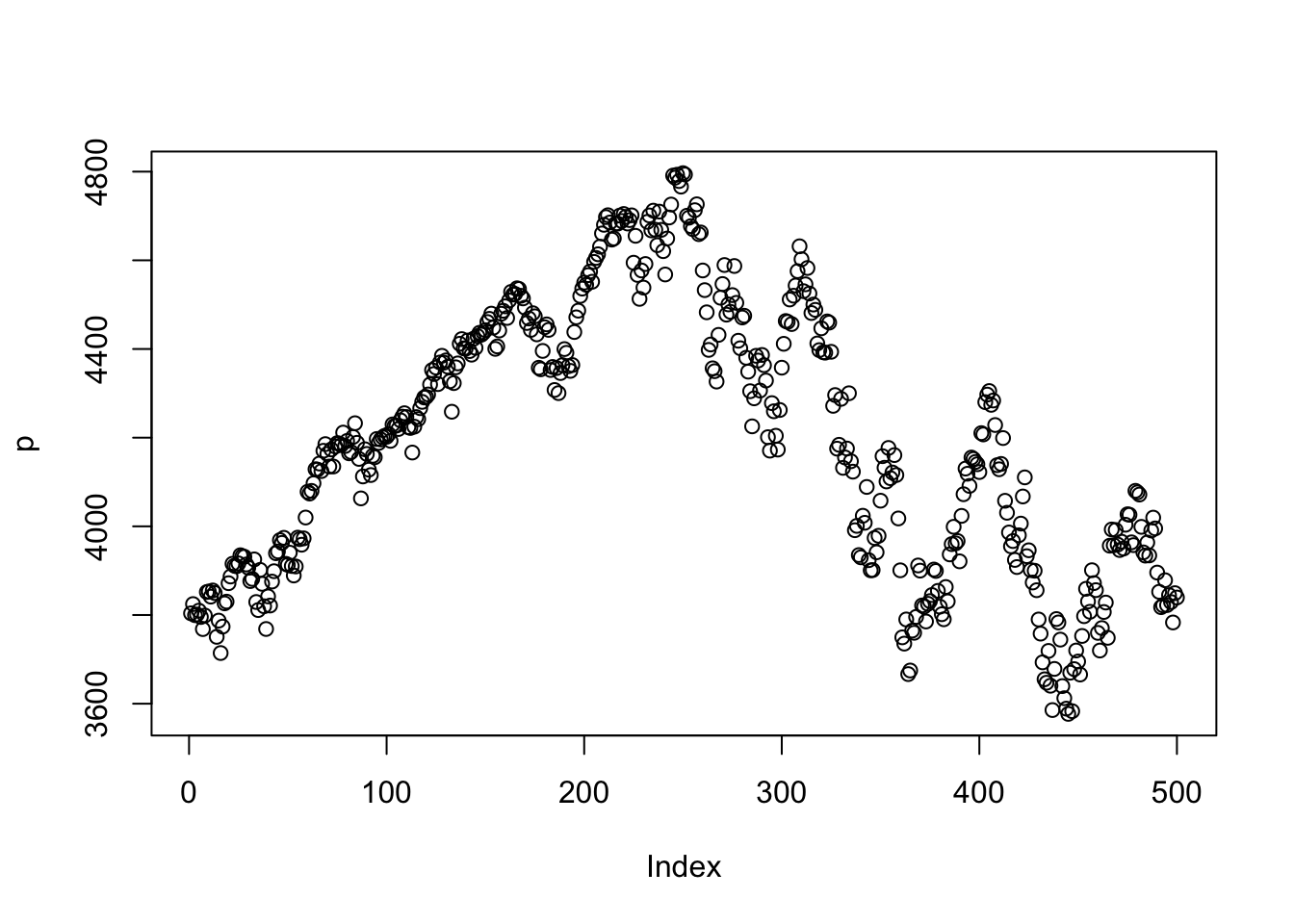Instructions:

• The colour of the title should be brown, with size 1.2;
• Plot $$y=x$$ in red, with line width 2;
• Draw $$x=2$$ and $$y=2$$ in dashed lines;
• Draw two polygons as shown above, one in blue and another in orange. There should be no boundaries for the orange one;
• Draw a green arrow from (8,2) to (6,2), the color should be set as green4;
• Draw a circle with centre (9,1) and radius 1.
• Add (2,6) and (4,4) to the plot, with coordinates clearly marked above/below the points;
• Add a violet, dashed box to the plot.

You can start by

plot(NULL, type="n", xlim=c(0,10), ylim=c(0,10), xlab="X Label",
ylab="Y Label", bty="n", asp=1)

This will generate an empty plot without boundary. Note that we set the aspect ratio (asp) to be 1 to make sure the circles and polygons are in the right shape. Aspect ratio refers to the ratio between the $$x$$ and $$y$$ coordinates. In some cases, you may need to specify the aspect ratio to ensure the plots are demonstrated accurately. For example, the circle in the above exercise should be tangent to $$x=2$$. An incorrect aspect ratio will twist this fact and may result in confusions.

# Answer
plot(NULL, type="n", xlim=c(0,10), ylim=c(0,10), xlab="X Label",
ylab="Y Label", bty="n", asp=1)
title(main="Practice Plot", col.main="brown", cex.main=1.2)  # add title
abline(0, 1, col="red", lwd=2)  # add line
polygon(c(1,1,3,3),c(6,8,6,8),col="blue")  # generate a polygon
polygon(c(6,8,6,8),c(10,10,8,8),col="orange", border = NA)  # generate a polygon
arrows(8,2,6,2, col="green4", lwd=3, length=0.1)  # add an arrow
points(c(2,6), c(4,4), pch=c(1,0))  # add points
text(c(2,6), c(4,4), label=c("(2,6)","(4,4)"), pos=c(1,3))  # add texts
box(lty=2, lwd=2, col="violet")  # add a box

## 8.3 High Level Functions

In this section, we discuss plots most commonly used in financial data analysis, generated by high level functions. We mainly use Return.csv generated in Chapter 7.

### 8.3.1 Return Distributions

In many financial theories, returns are usually assumed to be normally distributed. In reality, stock returns are usually heavy-tailed and skewed. To visualize the distribution of stock returns, we can use histogram. hist command in base R plot allow us to create histograms easily. Here, we plot the return distribution of Apple, from 2003 to 2022.

stkrt=read.csv("data/Return.csv", header=T)
hist(stkrt$AAPL, freq=F, main="Return Histogram - AAPL", xlab="Return", col="deepskyblue3", breaks=30) # histogram x=seq(-0.2,0.15,0.001) lines(x,dnorm(x, mean(stkrt$AAPL), sd(stkrt$AAPL)), lty=2, col="red", lwd=2) # normal density line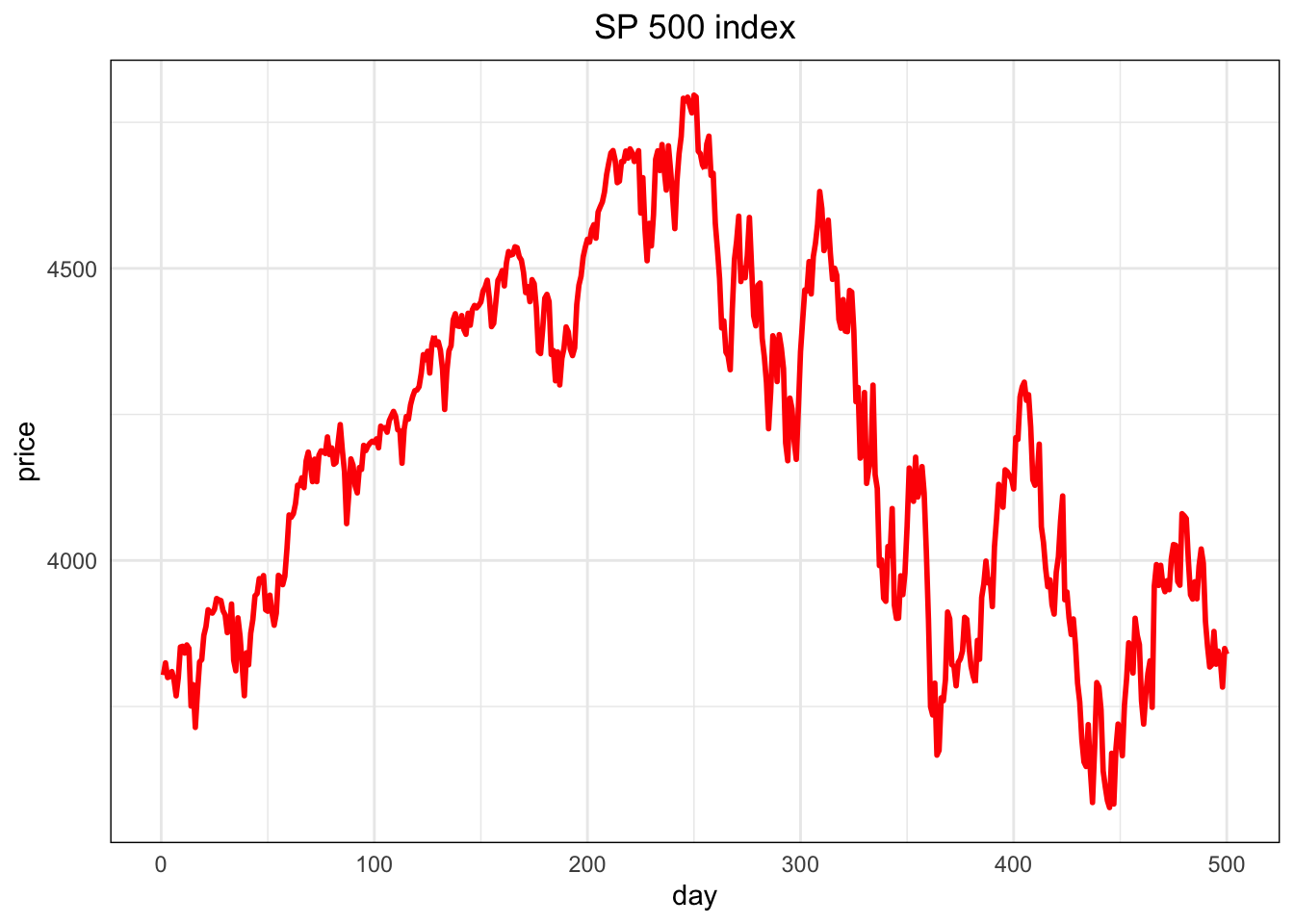freq=F displays histograms with density instead of frequency. This is more convenient if we want to compare the empirical distributions with the normal density. main="plot_name" sets the title of the plot and breaks=30 sets the number of bins. To add a normal density line, use lines command. The mean and standard deviation are set to match the sample mean and sample volatility. From the plot, we see that although the empirical distribution has a bell-shape, the returns have fat tails and are skewed. Returns around mean are more frequent than normal distribution, so Apple stock returns are not normally distributed. More rigorous ways of checking normality include Jarque-Bera test, Kolmogorov-Smirnov test, etc. Q-Q plot, discussed later in this book, is also a good way to visually check whether the normality assumption is violated. Another way to see the return distributions is the box plot. The upper and lower edges of the box are the 75th and 25th percentile, and the thick line in the middle represents the median. All points beyond the boundary of whiskers are classified as outliers. boxplot(stkrt$AAPL, main="Box Plot - AAPL", col="deepskyblue3", ylab="Return")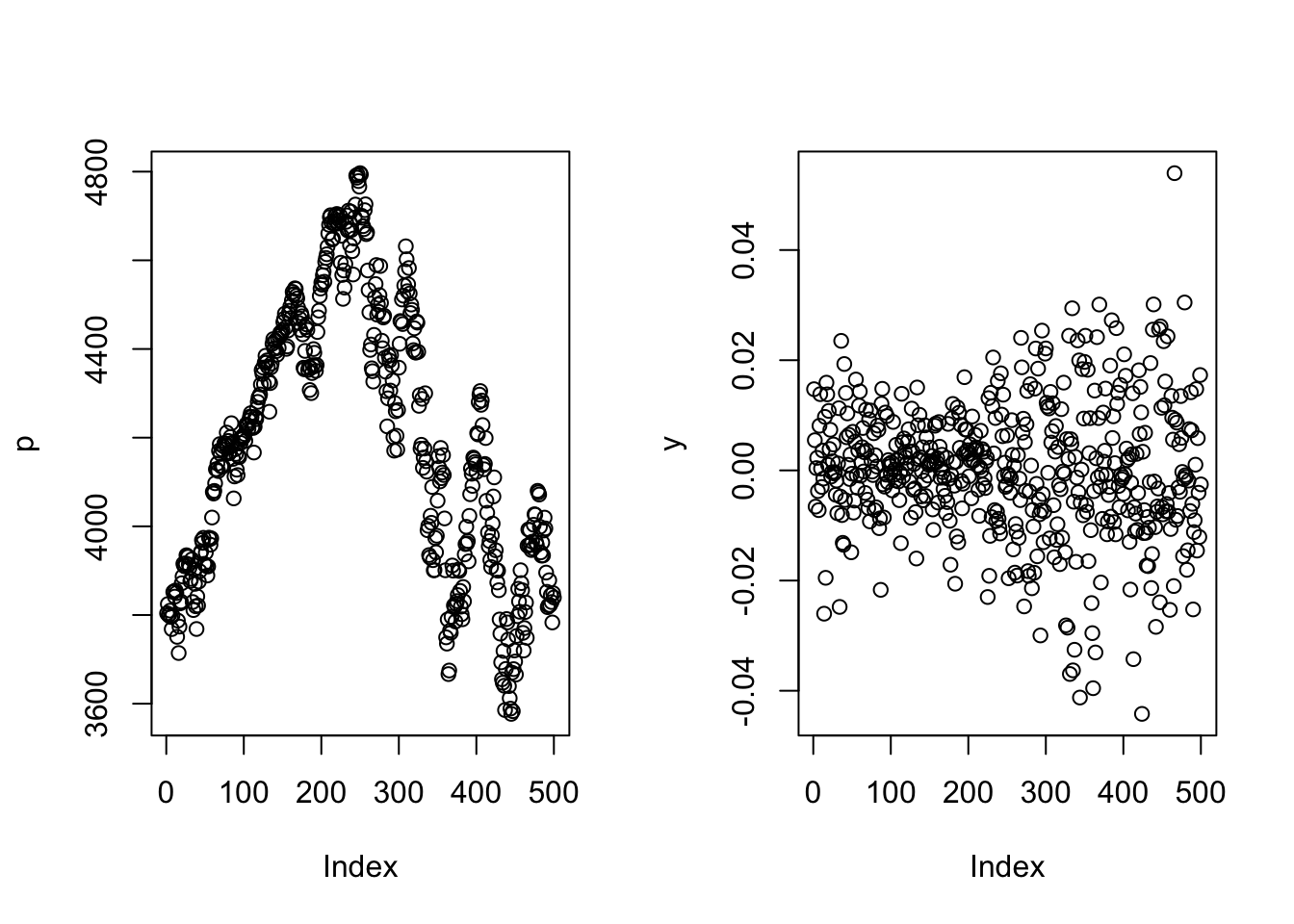We see a significant numbers of outlines in AAPL stock returns. This indicates extremely high/low returns are not rare. If you wish to make a horizontal box plot, add horizontal=T.

### 8.3.2 Time Series Plots

Time series is important for financial data analysis. A more comprehensive discussion for time series is in Chapter 8. For now, we will briefly discuss how to create time series plots. R itself has a time series class ts.

ts(data, start, end, frequency, ...)  # convert a numeric vector to a time series object
plot(ts)  # generate a time series plot

ts command converts a numeric vector into a time series object. Users can specify the time of the start and the end, and frequency stands for number of observations in one period of time. Apart from the time series data, date (time) is also an attribute of ts objects. For this reason, there is no need to include a date vector when plotting ts objects.

However, the property of ts can be undesirable in some cases, especially when we are dealing with daily data with small sample sizes. As stocks are not traded on weekends and holidays, missing values can be very problematic when R interpolates missing values. Time series plots can look bizarre as a result of interpolation, and you may wish to treat dates as discrete values depending on specific datasets. Therefore, we sometimes prefer to use plot(date_object, data) instead of plot(ts_object).

We illustrate this by using the stock returns of Apple from 2003 to 2022 using Return.csv. To plot the time series we will need to convert the date column in the dataset into a date class object. This can be completed using the as.Date() function. Note that as.Date() converts between character objects to date objects. As the dates in the dataset are numeric values, we need to convert numeric objects into character objects first. This can also be achieved by lubridate package (See Chapter 8).

stkrt$date=as.Date(as.character(stkrt$date), format = "%Y%m%d")
plot(stkrt$date, stkrt$AAPL, main="Daily Return - AAPL", xlab="Date", ylab="Return", type="l")
abline(h=0, lty=2, col="deepskyblue3")
idxmin=which(stkrt$AAPL==min(stkrt$AAPL))  # find the index which has the lowest return
idxmax=which(stkrt$AAPL==max(stkrt$AAPL))  # find the index which has the highest return
points(c(stkrt$date[idxmax], stkrt$date[idxmin]), c(stkrt$AAPL[idxmax], stkrt$AAPL[idxmin]),
col=c("red", "blue"), pch=16)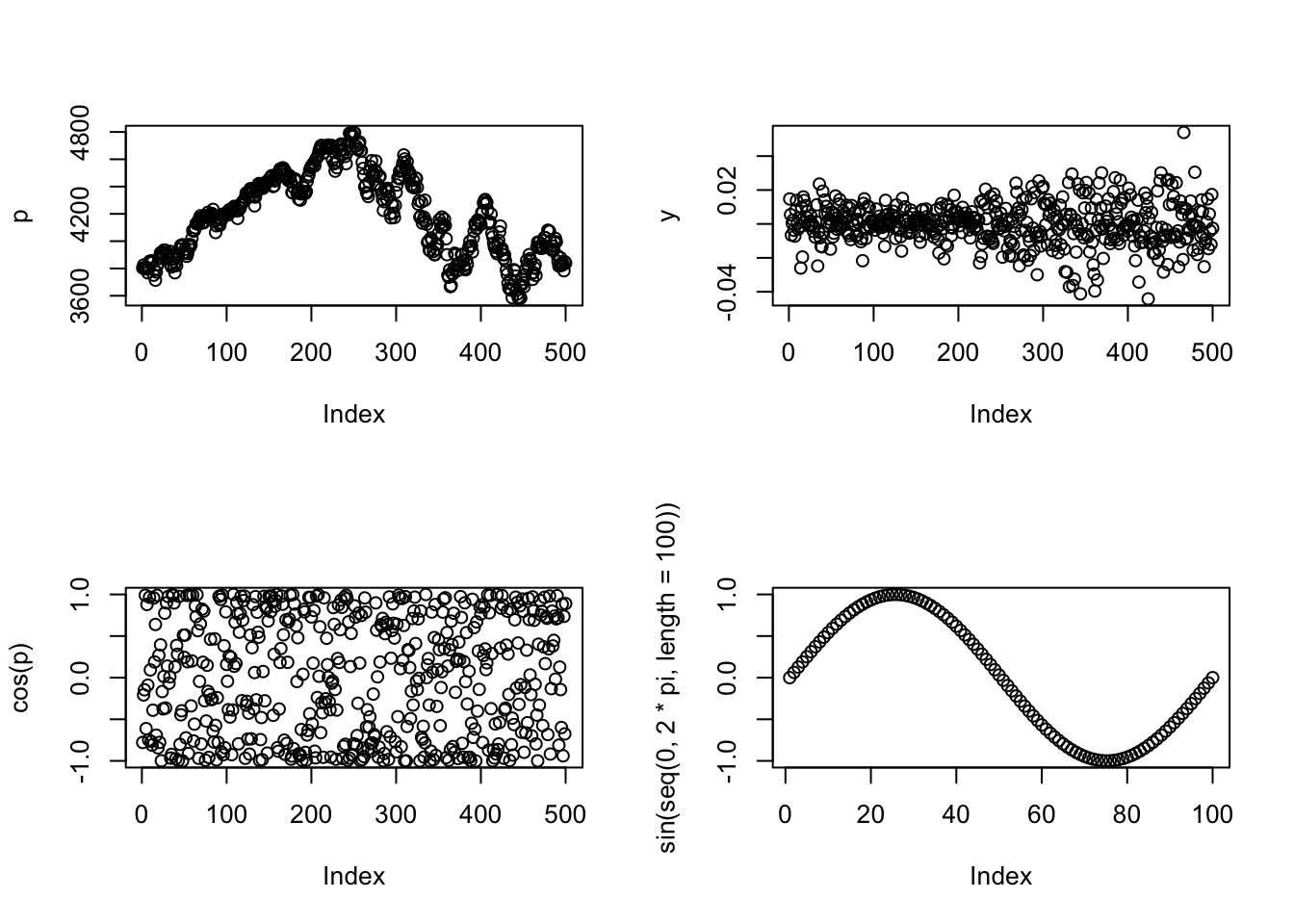which returns the index of the value which satisfies the given condition. With this we can easily locate the maximum and minimum of the given time series. We can see the most extreme values both occurred in 2008 during the Great Financial Crisis. If you wish to combine two sub-plots into one plot for a side-to-side comparison, use par(mfrow=c(# of rows, # of columns)). For example, to compare the stock returns of apple and GE, we use

par(mfrow=c(1,2))  # layout of sub-plots
plot(stkrt$date, stkrt$AAPL, main="Daily Return - AAPL", xlab="Date", ylab="Return",
type="l", ylim=c(-0.2, 0.2))
plot(stkrt$date, stkrt$GE, main="Daily Return - GE", xlab="Date", ylab="Return",
type="l", ylim=c(-0.2, 0.2))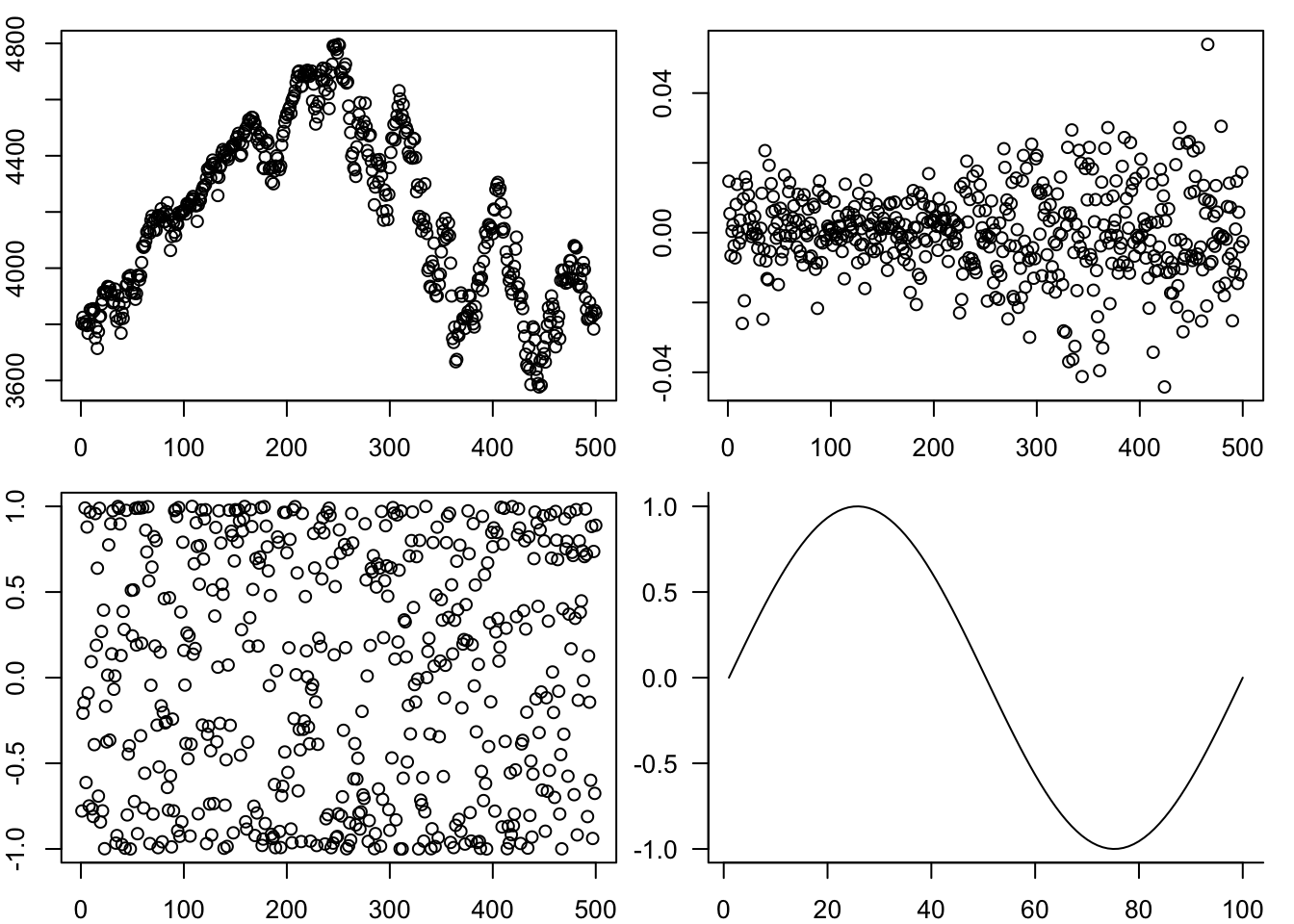From the plot, it is evident that GE returns are more cyclical than Apple over the entire horizon.

### 8.3.3 3D Plots

Univariate models have their limitations and multivariate extensions can be applied more widely for a portfolio of assets. Base R plots support creating 3D plots, and higher-dimensional data can be visualized with contour plots. Let’s consider the bivarite normal case where $$\mathbf{X}_1, \mathbf{X}_2$$ follow a bivariate normal distribution, i.e.,

$\mathbf{X}=\begin{pmatrix}\mathbf{X}_1\\\mathbf{X}_2\end{pmatrix}\qquad \mathbf{X}\mathbf\sim \mathcal{N}\begin{pmatrix}\mathbf{\mu}\\\mathbf{\Sigma}\end{pmatrix}$ with $\mathbf{\mu}=\begin{pmatrix}0\\0\end{pmatrix}\qquad \mathbf{\Sigma}=\begin{pmatrix}1&0.5\\0.5&1\end{pmatrix}$ To generate random variables that are bivariate normal, it is useful to load the mnormt package.

library(mnormt)

The built-in contour and persp command can produce basic contour plots and 3D surface plots.

x1=seq(-3, 3, 0.1)
x2=seq(-3, 3, 0.1)
mu=c(0, 0)  # mean
sigma=matrix(c(1, 0.5, 0.5, 1), nrow = 2)  # var-cov matrix
fx=function(x1, x2){dmnorm(cbind(x1, x2), mu, sigma)}  # bivariate function
y=outer(x1, x2, fx)
contour(x1, x2, y, main="Contour Plot", xlab="x1", ylab="x2")  # contour plot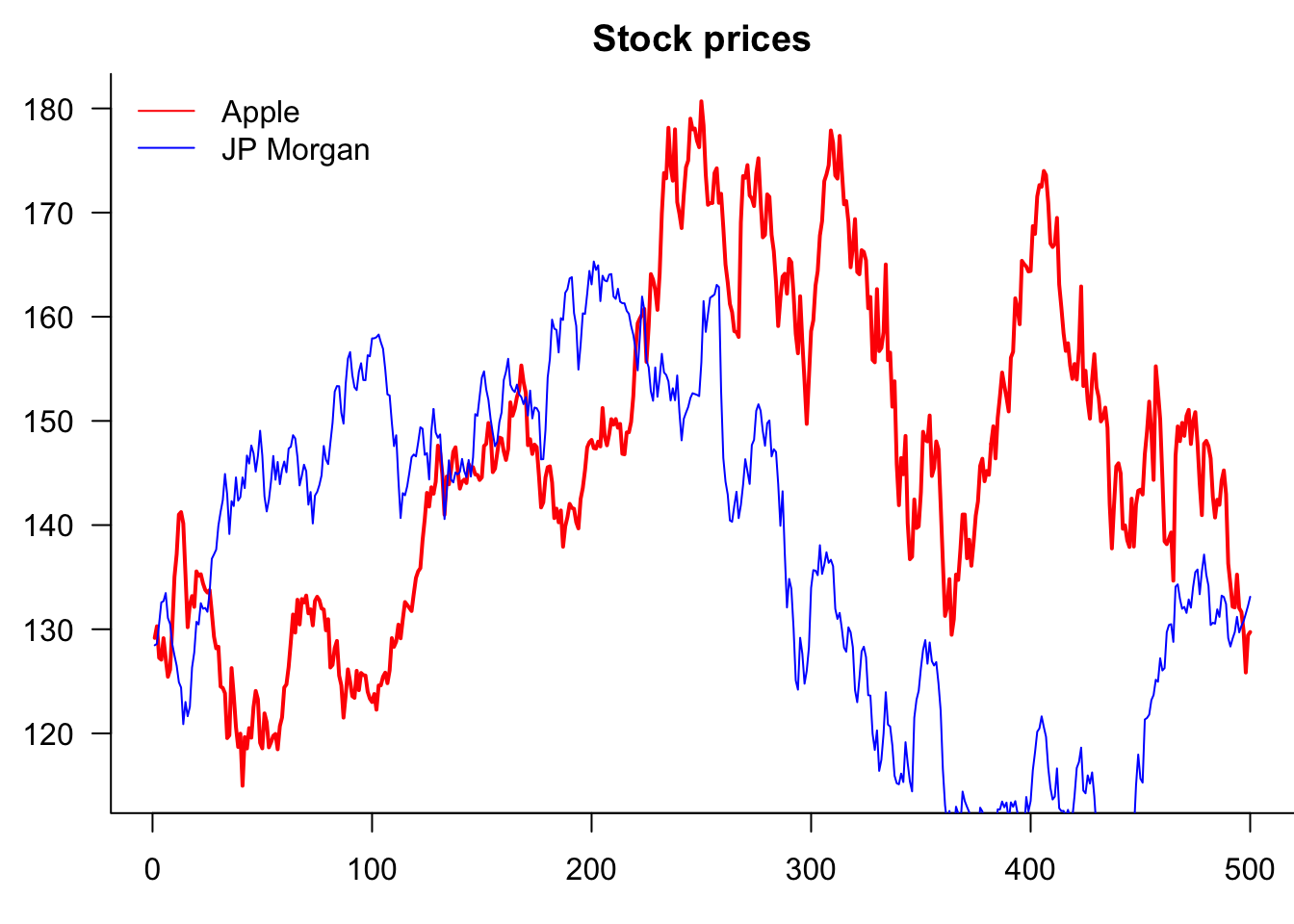persp(x1, x2, y, theta=-30, phi=15,
main="Bivariate Normal Surface")  # 3D plottheta and phi define angles of the viewing directions. Others arguments such as expand and r control how the plots are presented, and it is up to the users to select a set of appropriate values to produce nice plots. As a reminder, the length of $$\mathbf{X}_1, \mathbf{X}_2$$ should not be too long when using persp, otherwise it will take a long time to generate the plot and the plot might appear uniformly black if grids are included.

### 8.3.4 Other Plots

Base R supports several other plots, such as pie chart (pie(...)), bar chart (barplot(...)), scatter plot, etc. The commands are very similar as what we have discussed.

## 8.4 More Examples

In this section, we attempt to create several more sophisticated plots using the techniques discussed in previous sections. These plots are relevant if you want to highlight something or to make the plots visually appealing.

### 8.4.1 Normal vs t-distribution

We start by generating a plot comparing normal and t-distributions. t-distributions are heavy-tailed and are convenient to use when we need fat-tailed distributions. The following plot highlights the difference between normal and t-distributions.

x=seq(-3,3,0.01)
plot(x,dnorm(x),type="l", lty=1, lwd=2, main="Normal vs t-distribution",
xlab="x", ylab="Probability")  # normal density
for (i in 1:5){
lines(x,dt(x,i), lty=i+1, lwd=2)
}  # for degrees of freedom 1 to 5, plot the density line
legend("topleft", c("Normal", "t(1)", "t(2)", "t(3)", "t(4)", "t(5)"),
lty=1:6, lwd=2, cex=0.8, inset=c(0.01, 0.01))  # add legend

y=seq(-3, -2, 0.01)
z=seq(2, 3, 0.01)
lines(y, dnorm(y), lwd=5, col="red")
lines(z, dnorm(z), lwd=5, col="red")
lines(y, dt(y,1), lwd=5, col="blue")
lines(z, dt(z,1), lwd=5, col="blue")  # highlight tails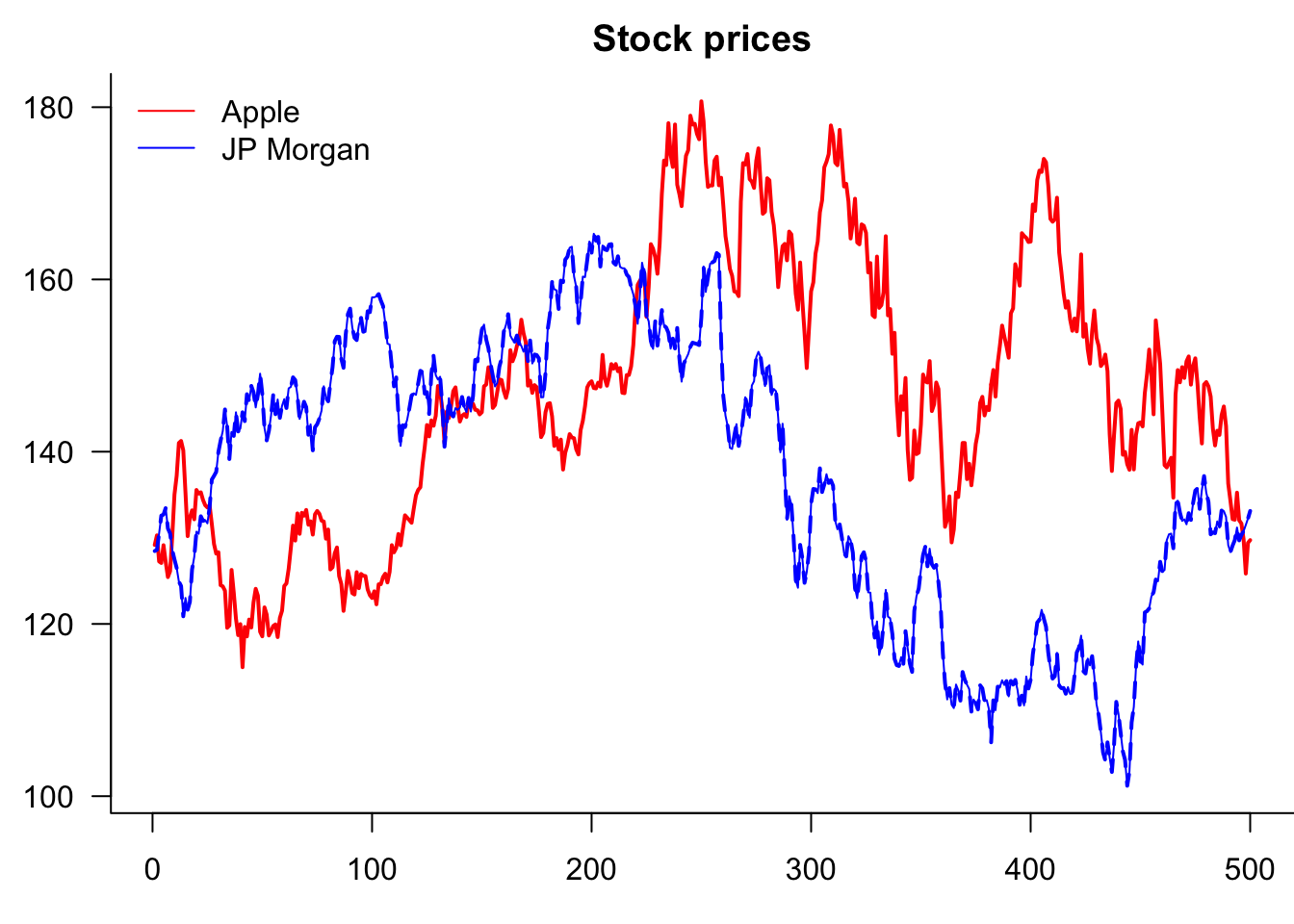for loops are significantly more efficient when similar elements need to be added to the same graph. In this example, we plotted the probability density function of t-distributions with degrees of freedom from 1 to 5. This can be achieved by using a for loop, as the only change involved is the degrees of freedom. The second half of the codes are intended to highlight the heavy tails of $$t(1)$$ distribution, using a line width of 5 (lwd=5).

### 8.4.2 Volatility Clusters

Volatility tend to come in cycles. Using sp500tr.csv, we examine the volatility clusters of S&P500 from 2003 to 2022. To visualize this, we make the following plot.

rt=read.csv("data/sp500tr.csv")
rt.df=as.data.frame(cbind(rt$date[-1], (rt$Close[-1]-rt$Close[-nrow(rt)])/rt$Close[-nrow(rt)]))
colnames(rt.df)=c("date", "rt")
rt.df$date=as.Date(as.character(rt.df$date), format="%Y%m%d")
rt.df=cbind(rt.df,as.numeric(format(rt.df$date,'%Y'))) colnames(rt.df)=c("date", "rt", "year") sd1=aggregate(rt.df$rt, list(rt.df$year), FUN=sd) # compute the standard deviation each year sd2=rbind(pmin(sd1[,2], mean(sd1[,2])), pmax(sd1[,2]-mean(sd1[,2]), 0)) barplot(sd2*100, names.arg = sd1[,1], main="Clusters in SP500 Volatility", ylab="Volatility", col=c("deepskyblue3", "red3"), border=NA) # stacked bar chart abline(h=0) abline(h=mean(sd1[,2])*100, lty=3, col="red3") text(x=16, y=1.2, "mean")This is a variant of stacked barplot, to highlight the years with above-average volatilities. Note that to add text to a bar chart, $$x$$ coordinates are categorical instead of numerical. For example, if you wish to add texts at year 2003, you should use $$x=1$$ instead of $$x=2003$$. ### 8.4.3 Empirical Density vs Normal The empirical cumulative distribution can be generated by ecdf. Using S&P500 return from 2003 to 2022, we have the following plot. rt.new=(rt$Close[-1]-rt$Close[-nrow(rt)])/rt$Close[-nrow(rt)]*100
plot(ecdf(rt.new), xlim=c(-5,5), col="deepskyblue3", lwd=3,
main="Empirical Density vs Normal", xlab= "Outcomes",
ylab="Cumulative Probability")  # empirical distribution plot
curve(pnorm(x, mean(rt.new), sd(rt.new)), from = -5, to=5,
abline(v=0.1, col="orange", lwd=2)
abline(v=-2.15, col="orange", lwd=2)
abline(v=2.6, col="orange", lwd=2)
text(-4, 0.1, "data higher\n than normal", cex=0.8)
text(1.3, 0.1, "data\n higher", cex=0.8)
text(4, 0.1, "data lower\n than normal", cex=0.8)
legend("topleft", legend=c("Returns", "Normal"), lwd=2, col=c("deepskyblue3","red"),
cex=0.8, bty="n", inset=c(0.01,0.02))  # add legend
polygon(c(-2.15,0.1,0.1,-2.15), c(par("usr"), par("usr"), par("usr"), par("usr")),
col=rgb(230, 180, 193, max = 255, alpha = 110), border=NA)  # shade a region
text(-0.5, 0.1, "data\n lower", cex=0.8)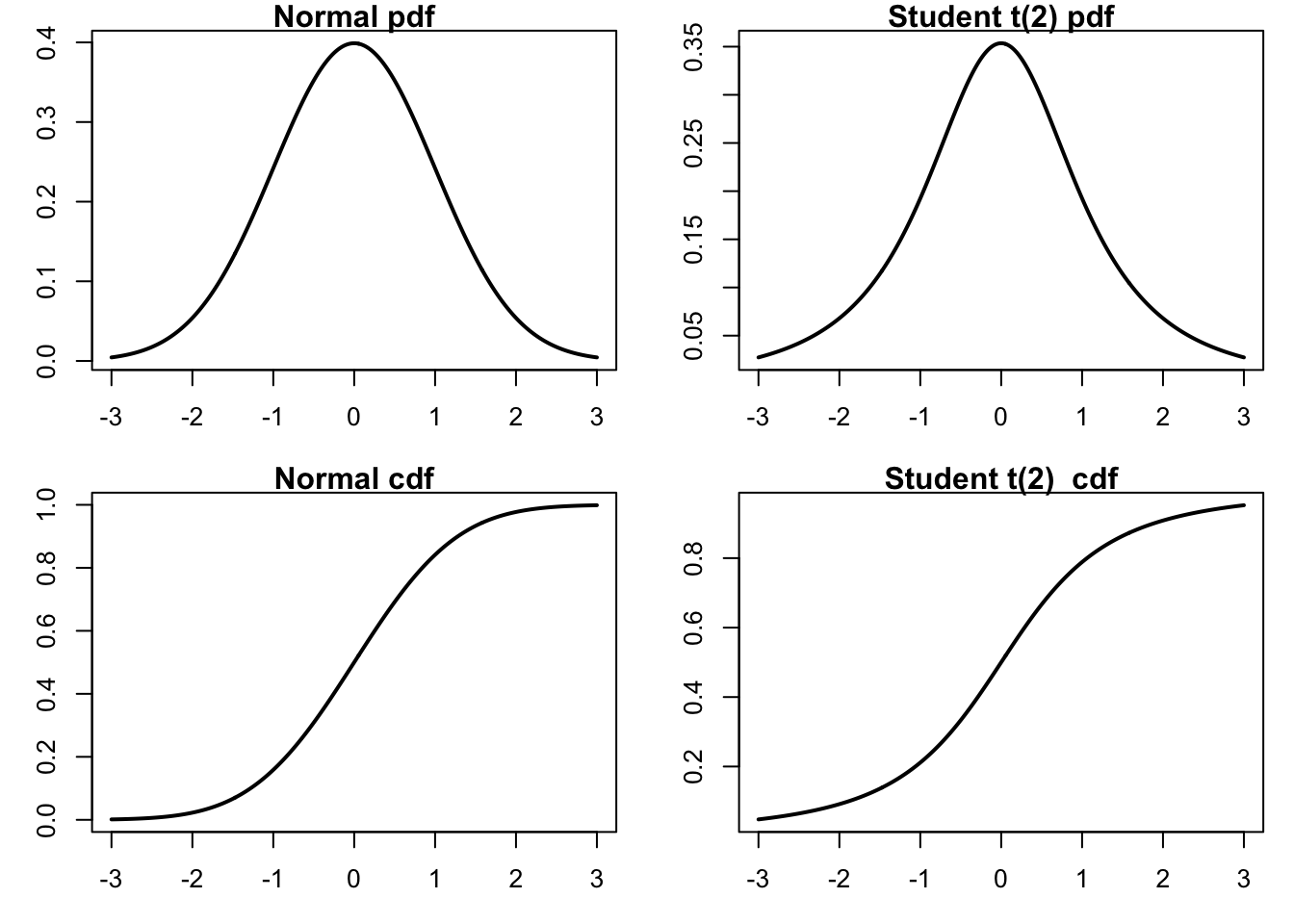curve() draws a function specified by the user. If you wish to shade a specific region in the plot, use polygon. This usually requires setting the colour to be transparent to make the lower layers visible, which can be customised by the rgb command.

### 8.4.4 VaR and Expected Shortfall

You may wish to shade the region under a curve. This again can be achieved by polygon function. To illustrate this, we compare VaR and Expected Shortfall in a single plot.

x=seq(-3, 3, 0.001)
y=seq(-3, -1.62, 0.001)
plot(x, dnorm(x), type="l", lwd=2, xlab="", ylab="",
main="VaR vs Expected Shortfall", ylim=c(0,1.1))
lines(y, dnorm(y)*10, lwd=2)
x1=x[(x>=-3)&(x<=-1.645)]  # select the tail region
y1=dnorm(x1)
x1=c(-3, x1, -1.645)  # add start and end points
y1=c(0, y1, 0)  # add start and end points
y2=y1*10
polygon(x1, y2, col="orange", border=NA)
polygon(x1, y1, col="brown", border=NA)
abline(v=-1.645, lwd=2, lty=2, col="black")
text(-0.5, 0.8, "Scale tail area to 1")For the polygon command to work we will have to add the first point and the last point to ensure the entire area is enclosed. This demonstrates how Expected Shortfall is related to VaR: ES is the expected loss conditional on VaR being violated, i.e., the orange region has an area of 1 in the plot.

## 8.5 Exporting Plots

After the plots are generated in R, you may wish to export and include them in your academic paper or slides. It is never a good idea to include screenshots in your writings, as they look unprofessional and are usually blurred. Instead, it is recommended to export plots from R by choosing appropriate image formats, such as PNG, JPEG, TIFF, SVG, EPS, PDF, etc.

In RStudio, it is possible to export the graphs from the bottom right preview panel. Click the Export icon, and you will be able to select through a range of image formats R supports, set directory, change file name, and specify the image size in the pop-up window. Alternatively, you can save plots with the following codes:

# save a plot in PDF
pdf("plot_name.pdf")  # specify the plot name and format
plot(...)  # generate your plot here
dev.off()  # close the plot device

Different image formats have different characteristics. PDF, or Portable Document Format, is most widely used in academic settings. PDF plots are in high resolution and can be zoomed in without loss of quality. SVG (Scalable Vector Graphics) and EPS (Encapsulated PostScript) are also vector-based, and are good alternatives to PDF. However, more commonly seen image formats such as PNG or JPEG are not scalable – PNG and JPEG plots are likely to be blurred and lose details once scaled up.

The selection of image formats depends on which word processor/text editor you are using. If you are using Word or PowerPoint, SVG is the recommended format. Importing SVG plots into Word and PowerPoint is simple: export the plot from R, and then in Word/PowerPoint, select Insert > Pictures > This Device. Navigate to the directory where the plot is saved, and then choose the one you want to insert. You can easily scale plots in Word/PowerPoint to proper sizes – SVG graphs are infinitely expandable without losing any resolution. They are also very suitable for webpages.

If you are writing with $$\LaTeX$$, PDF and EPS are preferred because $$\LaTeX$$ back-end does not support SVG directly. Plots can be easily imported using \includegraphics{filename} command with graphicx package loaded in the preamble. Although it is not impossible to include SVG plots in $$\LaTeX$$, there are prerequisites for them to work and can sometimes cause errors.

## 8.6ggplot2 and plotly

ggplot2 and plotly are powerful data visualization packages in R. They can significantly improve the aesthetics and quality of plots. plotly is specially intended for interactive graphs.

Compared with base R plots, ggplot commands are different. ggplot starts by calling ggplot(data, aes(...)), where data represents the dataset and aes() captures the variables. ggplot then draws the graph layer by layer, with a plus sign at the end of each line indicating a new layer is added. For example, geom_area(fill=..., alpha=...) shades the area under curve, where alpha is the degree of transparency. By default, ggplots have a grey background and white grids. This can be customized, with several themes available to choose from. Below is a time series plot of the S&P500 index in the past 20 years.

library(ggplot2)  # load the package first

rt$date=as.Date(as.character(rt$date),format = '%Y%m%d')  # convert into Date class

p=ggplot(rt, aes(x=date, y=Close)) +
geom_area(fill="deepskyblue3", alpha=0.4) +  # shade the area under the curve
geom_line(color="deepskyblue3", size=0.8) +  # set the colour and size of the line
theme_bw() +  # set theme of the graph
xlab("Date") +  # x-axis label
ylab("Index") +  # y-axis label
ggtitle("S&P500 Index (2003-2022)")  # plot title
pggplot2 can be used jointly with plotly. An interactive plot can be easily generated based on ggplot objects.

library(plotly)  # load the package first
ggplotly(p)

This plot allows you to interactively visualize the data. If you place the cursor on a data point, it will show explicitly the date and the index of each point.

The above plots are simple illustrations of how ggplot2 and plotly work. There are infinitely many good-looking plots you can generate with these packages. For more examples with reproducible codes, visit R Graph Gallary, a collection of hundreds of beautiful plots created with R.

## 8.7dygraphs

library(dygraphs)
lungDeaths <- cbind(mdeaths, fdeaths)
dygraph(lungDeaths) %>%
dySeries("mdeaths", label = "Male") %>%
dySeries("fdeaths", label = "Female") %>%
dyOptions(stackedGraph = TRUE) %>%
dyRangeSelector(height = 20)